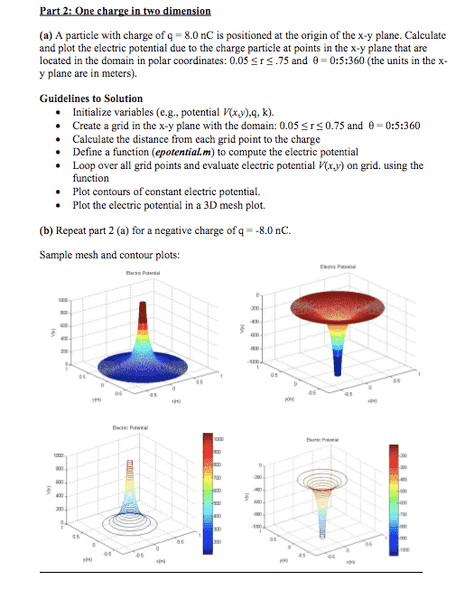# Need Help in MATLAB Contours

• MATLAB

Here is what I am working on.I know it may seem stupid, but I am very new to MATLAB.

I do not understand how to "create a grid in the x-y plane with domain r from .05 to .75
and theta 0:5:360"

I have created a "grid" using meshgrid...but I can't seem to wrap my head around doing it in polar

I have done this to create a meshgrid before:

Code:
x1=-0.5:0.01:-0.1;
x2=0.1:0.01:0.5;
x=[x1 x2];

y1=-0.5:0.01:-0.1;
y2=0.1:0.01:0.5;
y=[y1 y2];

[X,Y]=meshgrid(x,y);

Can someone help me extend this to Polar ?

As a matter of fact, I guess I do not understand most of this...

Why would I have to calculate the "distance to each point?" That is just r is it not?

wtf

Can anyone help me to interpret this correctly? I am about to go off the deep end here.

Yes, R is the distance from the charge to the point. You have to calculate it because MATLAB doesn't do polar grids. Do have to do the conversion from cartesian to polar coordinates yourself. Does this help?

-Kerry

## 1. What is MATLAB Contours?

MATLAB Contours is a feature in the MATLAB software that allows users to visualize 2D and 3D data through contour plots, which are graphical representations of data using lines or curves to connect points of equal value.

## 2. How do I plot contours in MATLAB?

To plot contours in MATLAB, you can use the contour or contourf functions, depending on whether you want the areas between the contours to be filled or not. These functions take in the data and plot the contours according to specified parameters.

## 3. Can I customize the contour plot in MATLAB?

Yes, you can customize the contour plot in MATLAB by specifying parameters such as the number of contour levels, color scheme, line style, and more. You can also add labels, titles, and legends to make the plot more informative and visually appealing.

## 4. How do I find the coordinates of a contour in MATLAB?

To find the coordinates of a contour in MATLAB, you can use the contourc function, which returns the contour matrix. This matrix contains the x and y coordinates of each point on the contour, as well as the contour level.

## 5. Can I combine multiple contour plots in MATLAB?

Yes, you can combine multiple contour plots in MATLAB using the hold on and hold off commands. These commands allow you to plot multiple graphs on the same figure, so you can easily compare and analyze different data sets.